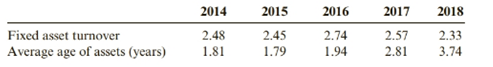Chapter 7, Problem 61E### Cornerstones of Financial Accounti...

4th Edition
Jay Rich + 1 other
ISBN: 9781337690881

#### Solutions

Chapter
Section### Cornerstones of Financial Accounti...

4th Edition
Jay Rich + 1 other
ISBN: 9781337690881
Textbook Problem
5 views

# Analyze Fixed AssetsTabor Industries is a technology company that operates in a highly competitive environment. In 2016, management had significantly curtailed its capital expenditures due to cash flow problems. Tabor reported the following information for 2019:â€¢ Net fixed assets (beginning of year), \$489,000â€¢ Net fixed assets (end of year), \$505,000â€¢ Net sales, \$1,025,000â€¢ Accumulated depreciation (end of year), \$543,000â€¢ Depreciation expense, \$126,000An analyst reviewing Tabor’s financial history noted that Tabor had previously reported fixed asset turnover ratios and average age of its assets as follows:During this time frame, the industry average fixed asset turnover ratio is 2.46 and the industry average age of assets is 1.79 years.Required:1. Compute Tabor’s fixed asset turnover ratio for 2019.2. Compute the average age of Tabor’s fixed assets for 2019.3. CONCEPTUAL CONNECTION Comment on Tabor’s fixed asset turnover ratios and the average age of the fixed assets.

To determine

Concept introduction:

Fixed assets turnover ratio:

Fixed assets play a major in the contribution of revenue to the company and they are significant for the efficient and continuous operation of the day to the day business. Fixed assets turnover ratio is used to measure how efficiently the company is using its fixed assets to generate revenue and if the company is more efficient then the ratio will be higher.

Requirement 1:

Compute fixed assets turnover ratio for 2019 of Tabor industries.

Explanation

The formula to calculate fixed assets turnover ratio is:

Fixed assets turnover ratio
...

To determine

Concept introduction:

Average age of fixed assets:

In addition to fixed assets ratio another important calculation is the average age of the fixed assets because older assets are less efficient than the newer assets. This calculation the managers to determine the capital replacement policy and future capital expenditures.

Requirement 2:

To explain:

Compute the average age of fixed assets for 2019 of Tabor industries.

To determine

Concept introduction:

Fixed assets turnover ratio:

Fixed assets play a major in the contribution of revenue to the company and they are significant for the efficient and continuous operation of the day to the day business. Fixed assets turnover ratio is used to measure how efficiently the company is using its fixed assets to generate revenue and if the company is more efficient then the ratio will be higher.

Average age of fixed assets:

In addition to fixed assets ratio another important calculation is the average age of the fixed assets because older assets are less efficient than the newer assets. This calculation the managers to determine the capital replacement policy and future capital expenditures.

Requirement 3:

To explain:

To comment on fixed assets turnover ratio and the average age of the fixed assets of tabor industries.

### Still sussing out bartleby?

Check out a sample textbook solution.

See a sample solution

#### The Solution to Your Study Problems

Bartleby provides explanations to thousands of textbook problems written by our experts, many with advanced degrees!

Get Started

#### Find more solutions based on key concepts